# quick sort and more: 快速排序算法总结

[TOC]

[Algo]

• 选择基准项(pivot element, 一般取第一个元素为pivot)
• 把数组里所有小于pivot的移动到pivot左边, 大于pivot的移动到右边 ⇒ 此时pivot已经位于最终排序时的正确位置
• 对pivot左右两个数组分别递归进行快速排序

## quick partition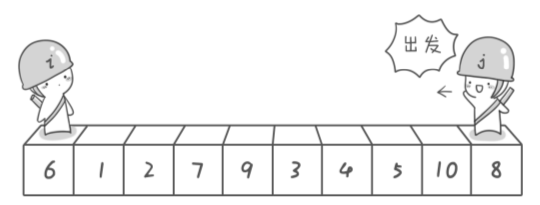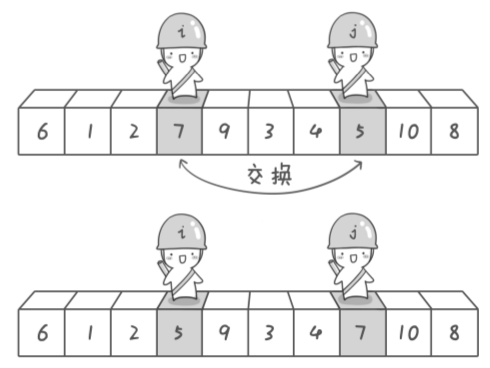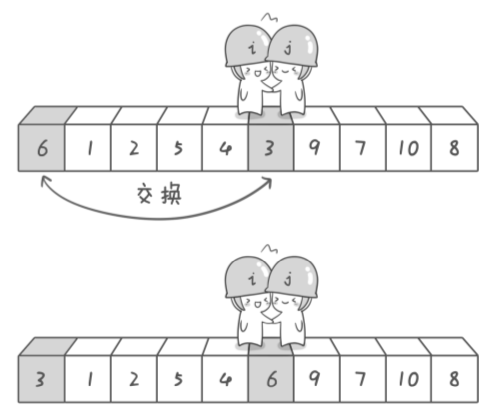java实现:

```int partition(int[] a, int lo, int hi){
int pivot = a[lo], i=lo, j=hi;
while(true){
for(;j>=lo && a[j]>=pivot;j--);// move j to a point where a[j]<pivot
for(;i<=hi && a[i]<=pivot;i++);// move i to a point where a[i]>pivot
if(i>=j) break;// break if i and j meets
swap(a, i++, j--);
}
swap(a, lo, j);// swap pivot with a[j]
return j;
}
```

• 让j先移动
• 最后pivot要和j交换而不是和i交换: 因为最后放在最左边的应该是一个小于pivot的数嘛
• 移动的时候别忘了需要加数组下标的边界检查(`i<=hi`, `j>=lo`)
• partition()的复杂度是线性的O(n)

## Knuth shuffle

qsort之所以快, 是因为每次都能够按照pivot分为大致同样长度的两个子数组(所以每次子问题的规模除以二), 所以复杂度为O(NlogN). 最坏情况下, 如果每次两个子数组中可能有一个长度为0, 那么每次子问题的规模只减少了1, 所以复杂度变成了quadratic O(N2).

```void shuffle(int[] a){
for(int K=0; K<a.length; K++)
swap(K, Random.nextInt(K+1));
}
```

## quick sort

```void qsort(int[] a, int lo, int hi){//recursive helper function
if(lo>=hi) return;
int p = partition(a, lo, hi);
qsort(a, lo, p-1);
qsort(a, hi, p+1);
}
void qsort(int[] a){
shuffle(a);
qsort(a, 0, a.length-1);
}
```

## quick select

```int findKth(int[] a, int k){
shuffle(a);
int lo=0, hi=a.length-1;
while(lo<hi){
int p = partition(a, lo, hi);
if(p==k) return a[k];
else if(p<k) lo=p+1;
else hi=p-1;
}
return a[k];
}
```

## 3-way qsort

qsort之前有个bug: 在数组里很多重复元素的时候, 效率会下降为O(N2). 原因是qsort没有好好处理重复元素的问题.

• a[lo,lt-1] < pivot
• a[lt, i-1] = pivot
• a[i,gt] = unseen
• a[gt+1, hi] > pivot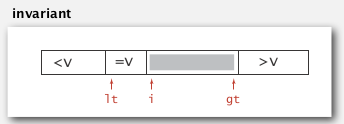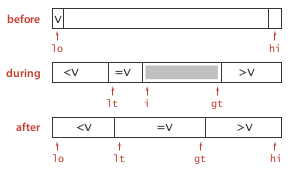```void qsort3way(int[] a, int lo, int hi){
if(hi<=lo) return;
int lt=lo, i=lo, gt=hi, pivot=a[lo];
while (i<=gt){// [i,gt] is unseen elements
if(a[i]==pivot) //a[lt,i-1] are elements == pivot
i++;
else if(a[i]>pivot) // a[gt+1, hi] are elements > pivot
swap(a, gt--, i);
else // a[lo, lt-1] are elements < pivot
swap(a, lt++, i++);
}
qsort3way(a, lo, lt-1);
qsort3way(a, gt+1, hi);
}
```

#### Part 5 of series «算法笔记»：

comments powered by Disqus